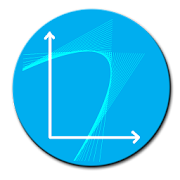# Parabola Solver - Lumos Educational App Store4.41
Price -fREE
\$0

#### DESCRIPTION:

Parabola solver helps the users to find different attributes of the parabola by providing the parabola equation in vertex , standard or intercept forms. I have included following : Vertex of the parabola Axis of symmetry Graph opens Focus Directrix Latus rectum equation Latus rectum length maximum or minimum value at vertex Domain Range X intercepts y intercept Added two more functions. now you can find the equation of the parabola if focus and vertex are given. you can also find the equation of the parabola if focus and directrix are given. User can input the values

#### OVERVIEW:

Parabola Solver is a free educational mobile app By .It helps students in grades HS practice the following standards HSG.GPE.A.2.

This page not only allows students and teachers download Parabola Solver but also find engaging Sample Questions, Videos, Pins, Worksheets, Books related to the following topics.

1. HSG.GPE.A.2 : Derive the equation of a parabola given a focus and directrix.

HS

#### STANDARDS:

HSG.GPE.A.2

Developer:

Software Version: 4.4

Category:

### RELATED APPSEdSearch WebSearch